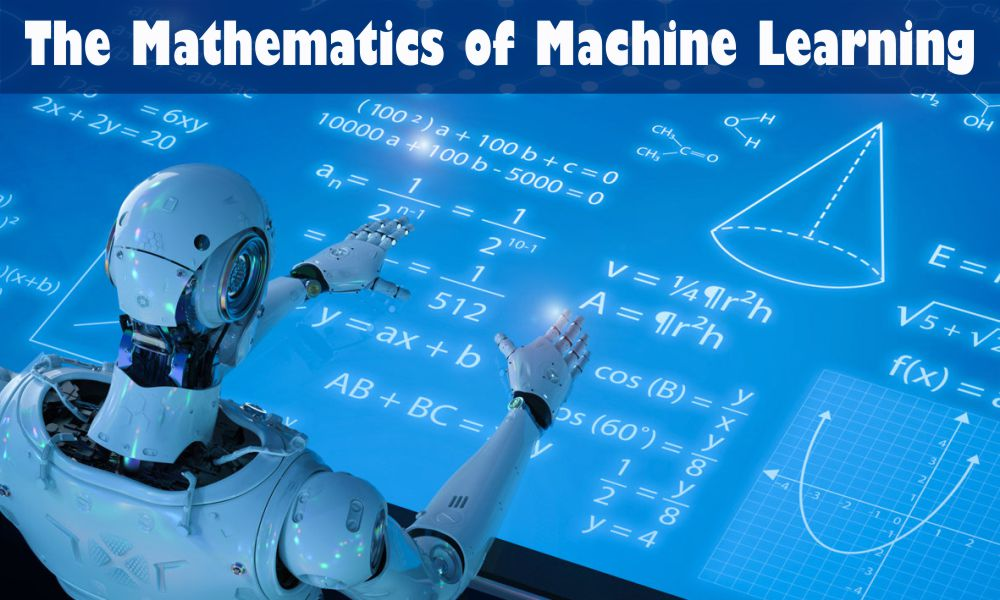# The Mathematics of Machine Learning

In current scenario, there has been an outstanding interest of people exploring into the world of data science and using Machine Learning (ML) practices to probe statistical regularities and build immaculate data-driven products. However, it’s also been observed that some actually lack the necessary mathematical awareness and background to get useful results.

Recently, there has been an upsurge in the availability of many easy-to-use machine and deep learning packages such as scikit-learn, Weka, Tensorflow etc. Machine Learning theory is a field that combines statistical, probabilistic, computer science and algorithmic aspects, arising from learning iteratively from data and finding hidden insights which can be used to build intelligent applications. Inspite of the immense possibilities of Machine and Deep Learning, a vast mathematical understanding of variety of these techniques is necessary for a better understanding of the inner workings of the algorithms and getting better results.

What Level of Maths Do You Need?

To be a Machine Learning Engineer / Scientist, the minimum level of mathematics is needed. Below are the some of the mathematics concept and their importance.• Linear Algebra:  Linear Algebra is very important aspect In Machine Learning. Topics such as Singular Value Decomposition, LU Decomposition, Principal Component Analysis (PCA), Eigen decomposition of a matrix, Matrix Operations, , Eigen Values & Eigen Vectors , Projections QR Factorization / Decomposition, Symmetric Matrices, Orthogonalization & Orthonormalization, , Norms and Vector Spaces are needed for  the better understanding of the optimization methods used for machine learning.
• Probability Theory and Statistics:  Machine Learning and Statistics are interconnected fields. Machine Learning can be defined as ‘doing statistics on a Mac’. Some of the fundamental Probability  and Statistical Theory needed for Machine Learning are Random Variables, Probability Rules & Axioms, Standard Distributions (Bernoulli, Binomial, Multinomial, Uniform and Gaussian), Combinatorics, Bayes’ Theorem, Variance and Expectation, Maximum a Posteriori Estimation (MAP) and Sampling Methods, Conditional and Joint Distributions, Moment Generating Functions, Maximum Likelihood Estimation (MLE), Prior and Posterior.
• Algorithms and Complex Optimizations:  For better understanding of the computational scalability and efficiency of Machine Learning and for exploiting sparsity in datasets algorithms and complex optimization plays an important role.  Knowledge of data structures (Binary Trees, Hashing, Heap, Stack, etc), Graphs, Dynamic Programming, Randomized & Sublinear Algorithm, Descents and Primal-Dual methods, Gradient/Stochastic are needed.
• Multivariate Calculus: Some of the important topics includes  Intergral  and Differential Calculus, Vector-Values Functions , Partial Derivatives, , Directional Gradient, Jacobian, Hessian, Laplacian and Lagrangian Distribution.
• Others:  Some of the Mathematics topics that are not described above also have importance in Machine Learning. These include Information Theory (Entropy, Information Gain), Real and Complex Analysis (Sets and Sequences, Topology, Metric Spaces, Single-Valued and Continuous Functions, Limits, Function Spaces and Manifolds.

So, have a strong base in mathematics in your first year of engineering from Best Engineering Faculty in Delhi NCR and take your first step to be a Machine Learning Engineer. Study Engineering from Top B.Tech College in Noida .

## 0 thoughts on “The Mathematics of Machine Learning”

1.Wwe Fighters says:

Thank you for sharing these free resources! Now more than ever people are in need of free and accessible entertainment. Anyone with children would greatly benefit from this list
Wwe Fighters

2.Dakshit says:

Very Informative

3.Kavya Mishra says:

nice blog

4.Alok srivastava says:

Thankz for telling the important of mathematics

5.nitesh says:

very informative blog and well explained